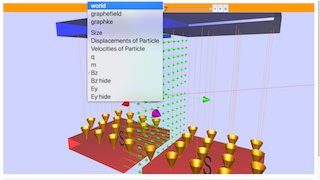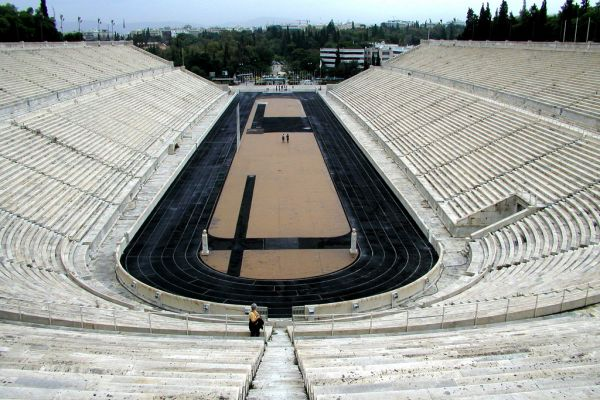Intro Page

Cyclotron Model

# Cyclotron Model Simulation

How the cyclotron works? http://en.wikipedia.org/wiki/Cyclotron and http://webphysics.davidson.edu/physlet_resources/bu_semester2/c13_cyclotron.html
The charged particles, injected near the center of the magnetic field Bz, accelerate only when passing through the gap between the electric field Ey electrodes with increase in kinetic energy. The perpendicular magnetic field Bz bends moving charges into a semicircular path between the magnets with no increase in kinetic energy. The magnetic field causes the charge to follow a half-circle that carries it back to the gap. While the charge is in the gap the electric field Ey is reversed, so the charge is once again accelerated across the gap. The cycle continues with the magnetic field in the dees continually bringing the charge back to the gap. Every time the charge crosses the gap it picks up speed. This causes the half-circles in the dees to increase in radius, and eventually the charge emerges from the cyclotron at high speed.
The combined motion is a result of increasing energy of the particles in electric field Ey and the magnetic field Bz forces the particles to travel in an increasing radius of the circle after each entry into the other magnetic field. This results in a spiral path of which the particles than emerged at a higher speed than when it was injected into the center of the magnetic field Bz.

Uses of the cyclotron http://en.wikipedia.org/wiki/Cyclotron
For several decades, cyclotrons were the best source of high-energy beams for nuclear physics experiments; several cyclotrons are still in use for this type of research.
Cyclotrons can be used to treat cancer. Ion beams from cyclotrons can be used, as in proton therapy, to penetrate the body and kill tumors by radiation damage, while minimizing damage to healthy tissue along their path.

Problems solved by the cyclotron http://en.wikipedia.org/wiki/Cyclotron
The cyclotron was an improvement over the linear accelerators
Cyclotrons accelerate particles in a spiral path. Therefore, a compact accelerator can contain much more distance than a linear accelerator, with more opportunities to accelerate the particles.

Cyclotrons produce a continuous stream of particles at the target, so the average power is relatively high.
The compactness of the device reduces other costs, such as its foundations, radiation shielding, and the enclosing building
This cyclotron model has:
Main view:
• 2 Magnets to provide for the magnetic field Bz to bend the moving charged particles in half circular path
• A gap between the 2 magnets in the y direction
• An alternating electric field Ey to accelerate the charge particle
• a charged particle.

### Credits:

The Cyclotron Model was created by Fu-Kwun Hwang, customized by Loo Kang Wee using the Easy Java Simulations (EJS) version 4.2 authoring and modeling tool.  An applet version of this model is available on the NTNU website < http://www.phy.ntnu.edu.tw/ntnujava/ >.

You can examine and modify this compiled EJS model if you run the model (double click on the model's jar file), right-click within a plot, and select "Open EJS Model" from the pop-up menu.  You must, of course, have EJS installed on your computer.  Information about EJS is available at: <http://www.um.es/fem/Ejs/> and in the OSP comPADRE collection <http://www.compadre.org/OSP/>

Exercises

exercises by lookang: adapted from http://webphysics.davidson.edu/physlet_resources/bu_semester2/c13_cyclotron.html

The building of the cyclotron model is based on a optional activity in http://www.opensourcephysics.org/items/detail.cfm?ID=8984 Charge in Magnetic Field Model written by Fu-Kwun Hwang edited by Robert Mohr and Wolfgang Christian

The learning from this optional activity demonstrate student's learning in performance tasks. 5 stars!

refer to http://www.phy.ntnu.edu.tw/ntnujava/index.php?topic=1972.0 for Charge Particle in Magnetic Field B Java Applet in 3D

Prior Knowledge required

charged particles

electric field & magnetic field

Engage

1. Early years scientists accelerate particle in linear accelerators but they face a problem of the need for a long linear path to accelerate the particle. Can you think of a way to reduce the need for a long path?

hint: look at the running track of a stadium, can you think of a way to bend the particle with the magnetic field and accelerate with electric field?After some discussions, students can share their ideas through oral/verbal presentation.

Teacher can praise some of the ideas and point them to Ejs as a means to test out their ideas using this Ejs simulation codes as templates for implementation.

Explore

1. Explore the simulation, this simulation is designed with a charge particle in a system of magnetic fields in z direction.

2 The play button runs the simulation, click it again to pause and the reset button brings the simulation back to its original state.

3 select Bz =0 (key in the value 0 follow by "enter" on keyboard), Ey =0, vy = 60, and play the simulation. Notice that the path of the particle in a straight line in the y direction. What is the physics principle simulatted here.

hint: newton's 1st law

4 reset the simulation.

5 using the default values(Bz =1, Ey=0, Vy=60), play the simulation. what did you observe? explain the motion in terms of the influences of magnetic field (assume gravitational effect can be neglected)

6 explore the slider x, y, and z. what do these sliders control?

7 explore the slider vx, vy, and vz. what do these sliders control?

8 by leaving the cursor on the slider, tips will appear to give a description of the slider. you can try it the following sliders such as the charge q, mass m, radius of dee(magnets) R.

9 there are some values radius of circular path r, kinetic energy of particle KE, resultant velocity vr and resultant force F on the m.

10 vary the simulation and get a sense of what it does.

11 reset the simulation

12 using the values(Bz =1, Ey=0, Vy=60, Ey =10. observe the difference in the introduction of Ey in the gaps.

13 notice that the Ey field is alternating, explain the purpose of this Ey in this simulation.

14 propose the logic deployed by this simulation to time the switching of Ey. Can you think of other swtiching logic?

15 note the first time the charge crosses the whole gap its kinetic energy increases by an amount ΔK. determine this value from looking at the value bar of KE, you may select the checkbox to view the scientific graph of KE vs t.

answer: 2421.4-2021.5 = 399.9 ≈ 400 J

16What is the change in kinetic energy associated with just moving in each half-circle in a dee (the magnetic field).

hint: look at the value bar of KE, you may select the checkbox to view the scientific graph of KE vs t.

Explain:

16 explain why this it is so?

hint: In the dee(magnetic field) the force on the charge comes from the magnetic field, so the force is perpendicular to the velocity. The speed, and hence the kinetic energy, stays constant, so the change is zero.

17 The first time the charge crosses the gap its kinetic energy increases by an amount ΔK say 400 J. Assuming the electric field in the gap is the same magnitude at all times but in opposite direction to earlier time, what is the change in kinetic energy the second time the charge crosses the gap?

hint: 2819.5-2421.4 = 398.1 ≈ 400 J

Elaborate

18 suggest with reason why the values for 15 and 17 are not exactly the same

hint: look at the value of vx

answer: the exiting from magnetic field causes the vx to be slightly bigger than 0, thus the resultant velocity is increased very slightly.

Evaluate:

19 A scientist ask a question "To increase the speed of the particles when they emerge from the cyclotron. Which is more effective, increasing the electric field Ey=-Vy/dy across the gap or increasing the magnetic field Bz in the dees? " play the simulation for different initial condition and design an experiment with tables of values to record systematically, determine what is the more "effective" method. State your assumptions made.

hint: assumption is outside physical radius of dee = R is fixed.

the start velocity vy =0

the start x = 0

Note that whatever the magnitudes of the fields the final half-circle the charge passes through in the dee has a radius approximately equal to R, the radius of the dee itelf. The radius of the circular path of a charged particle in a magnetic field is:

N2L: F = ma

circular: v.B.q = m.v^2/r

r = mv/Bq.

In this case the speed of the particle is RBq/m = v

Therefore the final kinetic energy is:

KE = 1/2 mv2 = 1/2. m. (RBq/m)^2 = 1/2. R^2q^2B^2/m

Have Fun!

This email address is being protected from spambots. You need JavaScript enabled to view it.

### Translations

Code Language Translator Run### Software Requirements

SoftwareRequirements

 Android iOS Windows MacOS with best with Chrome Chrome Chrome Chrome support full-screen? Yes. Chrome/Opera No. Firefox/ Samsung Internet Not yet Yes Yes cannot work on some mobile browser that don't understand JavaScript such as..... cannot work on Internet Explorer 9 and below

### CreditsFu-Kwun Hwang - Dept. of Physics, National Taiwan Normal Univ. remixed by lookang (This email address is being protected from spambots. You need JavaScript enabled to view it.); Fremont Teng; Loo Kang Wee

[text]

## Cyclotron Simulator JavaScript Simulation Applet HTML5

### Instructions

#### Combo Box and Functions

Toggling the combo box will select their respective functions.

Selecting world, graphefield and graphke will toggle their respective world view.

(world)

(graphefield)

(graphke)

Toggling through Size, Displacements of Particle, Velocities of Particle,
q and m sliders.

Toggling the other two will toggle their respective visibility.

(Bz show)

(Ey show)

#### Toggling Full Screen

Double click anywhere on the screen to toggle full screen.
Note that it only works if the simulation is Paused.

#### Play/Pause, Step and Reset Buttons

Plays/Pauses, steps and resets the simulation respectively.

Research

[text]

### Video

1. https://youtu.be/xO4Dtz9vkiI    An Introduction to the K500 Cyclotron by nsclmedia

### end faq